# nyquist

Nyquist plot of frequency response

## Syntax

``nyquist(sys)``
``nyquist(sys1,sys2,...,sysN)``
``nyquist(sys1,LineSpec1,...,sysN,LineSpecN)``
``nyquist(___,w)``
``[re,im,wout] = nyquist(sys)``
``````[re,im,wout] = nyquist(sys,w)``````
``````[re,im,wout,sdre,sdim] = nyquist(sys,w)``````

## Description

example

````nyquist(sys)` creates a Nyquist plot of the frequency response of a dynamic system model `sys`. The plot displays real and imaginary parts of the system response as a function of frequency. `nyquist` plots a contour comprised of both positive and negative frequencies. The plot also shows arrows to indicate the direction of increasing frequency for each branch. `nyquist` automatically determines frequencies to plot based on system dynamics.If `sys` is a multi-input, multi-output (MIMO) model, then `nyquist` produces an array of Nyquist plots, each plot showing the frequency response of one I/O pair.If `sys` is a model with complex coefficients, then the positive and negative branches are not symmetric.```

example

````nyquist(sys1,sys2,...,sysN)` plots the frequency response of multiple dynamic systems on the same plot. All systems must have the same number of inputs and outputs.```

example

````nyquist(sys1,LineSpec1,...,sysN,LineSpecN)` specifies a color, line style, and marker for each system in the plot.```

example

````nyquist(___,w)` plots system responses for frequencies specified by `w`.If `w` is a cell array of the form `{wmin,wmax}`, then `nyquist` plots the response at frequencies ranging between `wmin` and `wmax`.If `w` is a vector of frequencies, then `nyquist` plots the response at each specified frequency. The vector `w` can contain both negative and positive frequencies.You can use `w` with any of the input-argument combinations in previous syntaxes.```

example

````[re,im,wout] = nyquist(sys)` returns the real and imaginary parts of the frequency response at each frequency in the vector `wout`. The function automatically determines frequencies in `wout` based on system dynamics. This syntax does not draw a plot.```

example

``````[re,im,wout] = nyquist(sys,w)``` returns the response data at the frequencies specified by `w`.If `w` is a cell array of the form `{wmin,wmax}`, then `wout` contains frequencies ranging between `wmin` and `wmax`.If `w` is a vector of frequencies, then `wout` = `w`. ```

example

``````[re,im,wout,sdre,sdim] = nyquist(sys,w)``` also returns the estimated standard deviation of the real and imaginary parts of the frequency response for the identified model `sys`. If you omit `w`, then the function automatically determines frequencies in `wout` based on system dynamics.```

## Examples

collapse all

Create the following transfer function and plot its Nyquist response.

$\mathit{H}\left(\mathit{s}\right)=\frac{2{\mathit{s}}^{2}+5\mathit{s}+1}{{\mathit{s}}^{2}+2\mathit{s}+3}$.

```H = tf([2 5 1],[1 2 3]); nyquist(H)```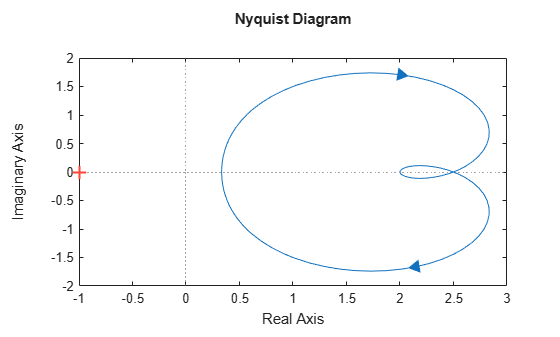The `nyquist` function can display a grid of M-circles, which are the contours of constant closed-loop magnitude. M-circles are defined as the locus of complex numbers where the following quantity is a constant value across frequency.

$\mathit{T}\left(\mathit{j}\omega \right)=|\frac{\mathit{G}\left(\mathit{j}\omega \right)}{1+\mathit{G}\left(\mathit{j}\omega \right)}|$.

Here, ω is the frequency in radians/`TimeUnit`, where `TimeUnit` is the system time units, and G is the collection of complex numbers that satisfy the constant magnitude requirement.

To display the grid of M-circles, right-click in the plot and select Grid. Alternatively, use the `grid` command.

`grid on`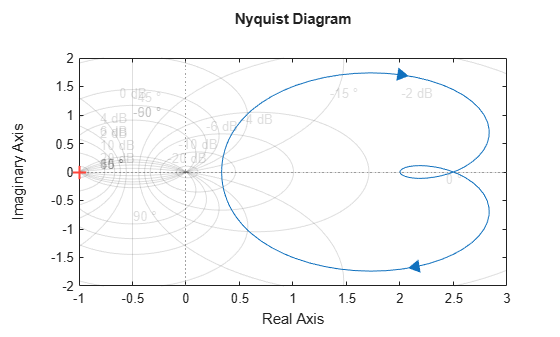Create a Nyquist plot over a specified frequency range. Use this approach when you want to focus on the dynamics in a particular range of frequencies.

```H = tf([-0.1,-2.4,-181,-1950],[1,3.3,990,2600]); nyquist(H,{1,100})```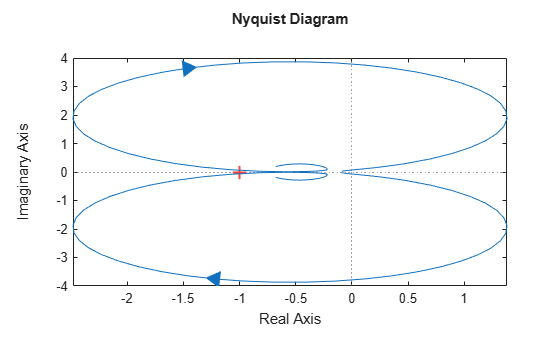The cell array `{1,100}` specifies a frequency range [1,100] for the positive frequency branch and [–100,–1] for the negative frequency branch in the Nyquist plot. The negative frequency branch is obtained by symmetry for models with real coefficients. When you provide frequency bounds in this way, the function selects intermediate points for frequency response data.

Alternatively, specify a vector of frequency points to use for evaluating and plotting the frequency response.

```w = 1:0.1:30; nyquist(H,w,'.-')````nyquist` plots the frequency response at the specified frequencies.

Compare the frequency response of several systems on the same Nyquist plot.

Create the dynamic systems.

```rng(0) sys1 = tf(3,[1,2,1]); sys2 = tf([2 5 1],[1 2 3]); sys3 = rss(4);```

Create a Nyquist plot that displays all systems.

```nyquist(sys1,sys2,sys3) legend('Location','southwest')```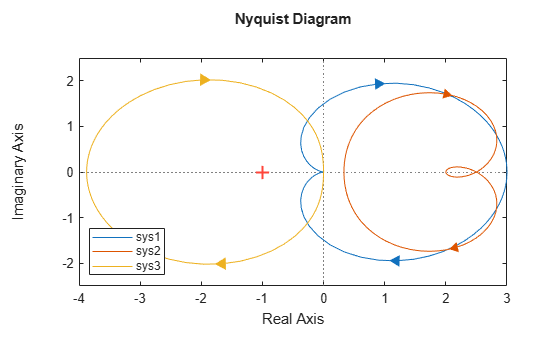Specify the line style, color, or marker for each system in a Nyquist plot using the `LineSpec` input argument.

```sys1 = tf(3,[1,2,1]); sys2 = tf([2 5 1],[1 2 3]); nyquist(sys1,'o:',sys2,'g')```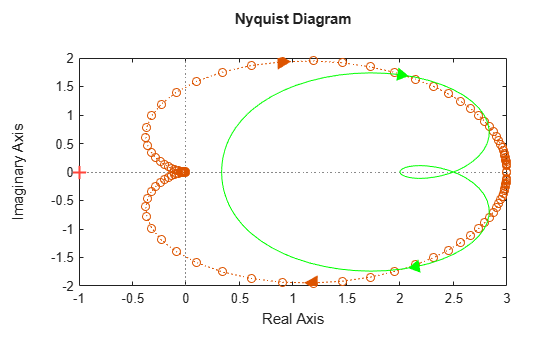The first `LineSpec`, `'o:'`, specifies a dotted line with circle markers for the response of `sys1`. The second `LineSpec`, `'g'`, specifies a solid green line for the response of `sys2`.

Compute the real and imaginary parts of the frequency response of a SISO system.

If you do not specify frequencies, `nyquist` chooses frequencies based on the system dynamics and returns them in the third output argument.

```H = tf([2 5 1],[1 2 3]); [re,im,wout] = nyquist(H);```

Because `H` is a SISO model, the first two dimensions of `re` and `im` are both 1. The third dimension is the number of frequencies in `wout`.

`size(re)`
```ans = 1×3 1 1 141 ```
`length(wout)`
```ans = 141 ```

Thus, each entry along the third dimension of `re` gives the real part of the response at the corresponding frequency in `wout`.

For this example, create a 2-output, 3-input system.

```rng(0,'twister'); H = rss(4,2,3);```

For this system, `nyquist` plots the frequency responses of each I/O channel in a separate plot in a single figure.

`nyquist(H)`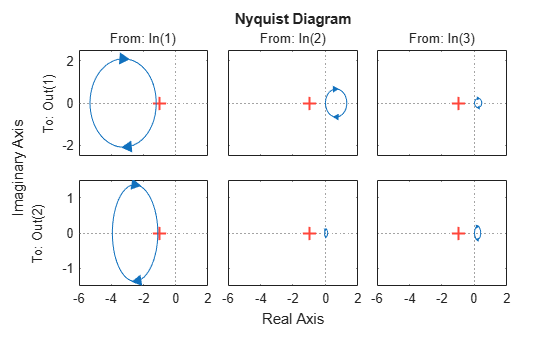Compute the real and imaginary parts of these responses at 20 frequencies between 1 and 10 radians.

```w = logspace(0,1,20); [re,im] = nyquist(H,w);```

`re` and `im` are three-dimensional arrays, in which the first two dimensions correspond to the output and input dimensions of `H`, and the third dimension is the number of frequencies. For instance, examine the dimensions of `re`.

`size(re)`
```ans = 1×3 2 3 20 ```

Thus, for example, `re(1,3,10)` is the real part of the response from the third input to the first output, computed at the 10th frequency in `w`. Similarly, `im(1,3,10)` contains the imaginary part of the same response.

Compute the standard deviations of the real and imaginary parts of the frequency response of an identified model. Use this data to create a 3σ plot of the response uncertainty.

Load the estimation data `z2`.

`load iddata2 z2;`

Identify a transfer function model using the data. Using the `tfest` command requires System Identification Toolbox™ software.

`sys_p = tfest(z2,2);`

Obtain the standard deviations for the real and imaginary parts of the frequency response for a set of 512 frequencies, `w`.

```w = linspace(-10*pi,10*pi,512); [re,im,wout,sdre,sdim] = nyquist(sys_p,w);```

`re` and `im` are the real and imaginary parts of the frequency response, and `sdre` and `sdim` are their standard deviations, respectively. The frequencies in `wout` are the same as the frequencies you specified in `w`.

Use the standard deviation data to create a 3σ plot corresponding to the confidence region.

```re = squeeze(re); im = squeeze(im); sdre = squeeze(sdre); sdim = squeeze(sdim); plot(re,im,'b',re+3*sdre,im+3*sdim,'k:',re-3*sdre,im-3*sdim,'k:') xlabel('Real Axis'); ylabel('Imaginary Axis');```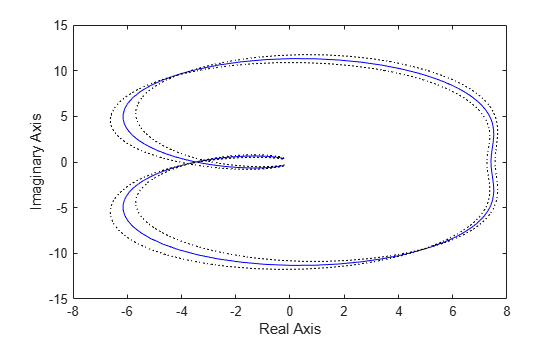Create a Nyquist plot of a model with complex coefficients and a model with real coefficients on the same plot.

```rng(0) A = [-3.50,-1.25-0.25i;2,0]; B = [1;0]; C = [-0.75-0.5i,0.625-0.125i]; D = 0.5; Gc = ss(A,B,C,D); Gr = rss(4); nyquist(Gc,Gr) legend('Complex-coefficient model','Real-coefficient model')```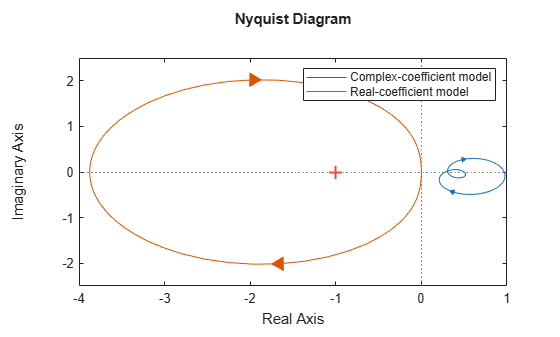The Nyquist plot always shows two branches, one for positive frequencies and one for negative frequencies. The arrows indicate the direction of increasing frequency for each branch. For models with complex coefficients, the two branches are not symmetric. For models with real coefficients, the negative branch is obtained by symmetry.

## Input Arguments

collapse all

Dynamic system, specified as a SISO or MIMO dynamic system model or array of dynamic system models. Dynamic systems that you can use include:

• Continuous-time or discrete-time numeric LTI models, such as `tf` (Control System Toolbox), `zpk` (Control System Toolbox), or `ss` (Control System Toolbox) models.

• Generalized or uncertain LTI models such as `genss` (Control System Toolbox) or `uss` (Robust Control Toolbox) models. (Using uncertain models requires Robust Control Toolbox™ software.)

• For tunable control design blocks, the function evaluates the model at its current value for both plotting and returning frequency response data.

• For uncertain control design blocks, the function plots the nominal value and random samples of the model. When you use output arguments, the function returns frequency response data for the nominal model only.

• Frequency-response data models such as `frd` models. For such models, the function plots the response at frequencies defined in the model.

• Identified LTI models, such as `idtf`, `idss`, or `idproc` models. For such models, the function can also plot confidence intervals and return standard deviations of the frequency response. See Create Nyquist Plot of Identified Model With Response Uncertainty.

If `sys` is an array of models, the function plots the frequency responses of all models in the array on the same axes.

Line style, marker, and color, specified as a string or vector of one, two, or three characters. The characters can appear in any order. You do not need to specify all three characteristics (line style, marker, and color). For example, if you omit the line style and specify the marker, then the plot shows only the marker and no line. For more information about configuring this argument, see the `LineSpec` input argument of the `plot` function.

Example: `'r--'` specifies a red dashed line

Example: `'*b'` specifies blue asterisk markers

Example: `'y'` specifies a yellow line

Frequencies at which to compute and plot frequency response, specified as the cell array `{wmin,wmax}` or as a vector of frequency values.

• If `w` is a cell array of the form `{wmin,wmax}`, then the function computes the response at frequencies ranging between `wmin` and `wmax`.

• If `w` is a vector of frequencies, then the function computes the response at each specified frequency. For example, use `logspace` to generate a row vector with logarithmically spaced frequency values. The vector `w` can contain both positive and negative frequencies.

If you specify a frequency range of [wmin,wmax] for your plot, then the plot shows a contour comprised of both positive frequencies [wmin,wmax] and negative frequencies [–wmax,–wmin].

Specify frequencies in units of rad/`TimeUnit`, where `TimeUnit` is the `TimeUnit` property of the model.

## Output Arguments

collapse all

Real part of the system response, returned as a 3-D array. The dimensions of this array are (number of system outputs)-by-(number of system inputs)-by-(number of frequency points).

Imaginary part of the system response, returned as a 3-D array. The dimensions of this array are (number of system outputs)-by(number of system inputs)-by-(number of frequency points).

Frequencies at which the function returns the system response, returned as a column vector. The function chooses the frequency values based on the model dynamics, unless you specify frequencies using the input argument `w`.

`wout` also contains negative frequency values for models with complex coefficients.

Frequency values are in radians per `TimeUnit`, where `TimeUnit` is the value of the `TimeUnit` property of `sys`.

Estimated standard deviation of the real part of the response at each frequency point, returned as a 3-D array. `sdre` has the same dimensions as `re`.

If `sys` is not an identified LTI model, `sdre` is `[]`.

Estimated standard deviation of the imaginary part of the response at each frequency point, returned as a 3-D array. `sdim` has the same dimensions as `im`.

If `sys` is not an identified LTI model, `sdim` is `[]`.

## Tips

• When you need additional plot customization options, use `nyquistplot` instead.

• Two zoom options that apply specifically to Nyquist plots are available from the right-click menu :

• Full View — Clips unbounded branches of the Nyquist plot, but still includes the critical point (–1, 0).

• Zoom on (-1,0) — Zooms around the critical point (–1, 0). To access critical-point zoom programmatically, use the `zoomcp` command. For more information, see `nyquistplot`.

• To activate data markers that display the real and imaginary values at a given frequency, click anywhere on the curve. The following figure shows a `nyquist` plot with a data marker.(Control System Toolbox) | |

### Topics

Introduced before R2006a

## SupportGet trial now